# Drawing a Perpendicular to a Line Through a Point Not on It

#### description

• Method using ruler and compasses
• Method using a ruler and a set-square

# Perpendicular to a Line Through a Point Not on It:

## A. By folding the paper:

• Draw a line MN on a paper. Take a point P anywhere outside the line.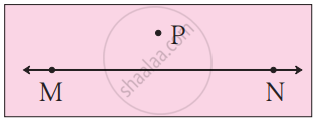• Keeping the line MN in view, fold the paper along the line MN.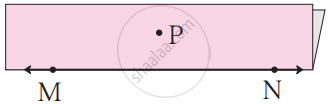• Now fold the paper through point P in such a way that the part of line MN on one side of the fold falls on the part of line MN on the other side of the fold.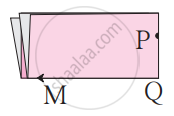• Unfold the paper. Name the point of intersection of the two folds Q. Draw the line PQ. This line falls on a fold in the paper.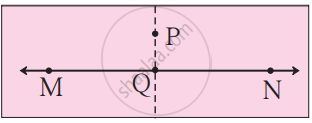Using a protractor, measure every angle formed at the point Q.
Line PQ is perpendicular to line MN.
Line PQ ⊥ line MN.

## A. Method using ruler and compasses:

Step 1: Given a line l and a point P not on it.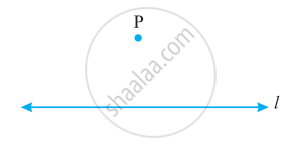Step 2: With P as a centre, draw an arc that intersects line l at two points A and B.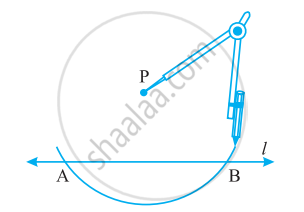Step 3: Using the same radius and with A and B as centers, construct two arcs that intersect at a point, say Q, on the other side.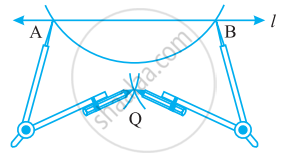Step 4: Join PQ. Thus, bar"PQ" is perpendicular to l.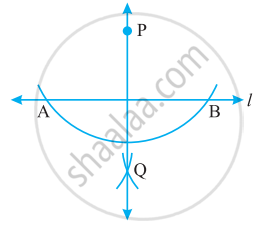## B. Method using a ruler and a set-square (An optional activity):

Step 1: Let l be the given line and P be a point outside l.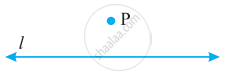Step 2: Place a set-square on l such that one arm of its right angle aligns along l.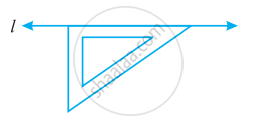Step 3: Place a ruler along the edge opposite to the right angle of the set-square.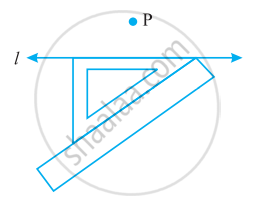Step 4: Hold the ruler fixed. Slide the set-square along the ruler till the point P touches the other arm of the set-square.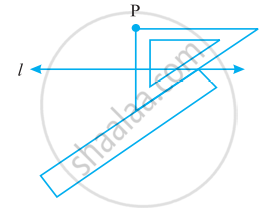Step 5: Join PM along the edge through P, meeting l at M.
Now, bar"PM" ⊥ l.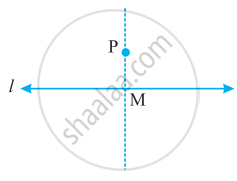If you would like to contribute notes or other learning material, please submit them using the button below.

### Shaalaa.com

Perpendicular to a Line Through a Point Not on It [00:03:13]
S
0%